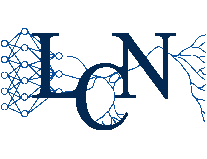Laboratory of Computational Neuroscience LCN - Brain Mind - I&C - Life Sciences - EPFL

# Neuronal Dynamics - Computational Neuroscience of Single Neurons

## Video Lectures on Single-Neuron Modeling:

by Wulfram Gerstner
• SEVERAL ONLINE -Lectures per week of about 10-20 Minutes each over 7 weeks (49 lectures total).

This course, designed for Physicists and mathematically trained Life Scientists focuses on modeling of single neurons . It covers classical topics of computational neuroscience such as the Hodgkin-Huxley models, and integrate-and-fire models, as well as modern state-of-the art approaches such as Generalized Linear Models or biophyiscal multi-compartment models.

Mathematical tools such as phase plane analysis, delta-function, and 'noise' are systematically introduced

Mathematical prerequisites:

• Calculus
• Differential Equations
• Probability